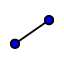# Trapezoid Median Discovery

## In this app below, quadrilateral ABCD is a trapezoid. Move points A, B, C, D around for a minute. Then answer the question that follows.

Complete the following sentence definition from what you see above: A trapezoid is a quadrilateral that has...

## AFTER MAKING YOUR TRAPEZOID ABOVE:

1. Use the DISTANCEtool to display the length of the trapezoid's parallel sides only. 1. Use the MIDPOINTtool to plot the midpoints of the 2 non-parallel-sides. 2. Use the SEGMENTtool to construct the segment that connects these midpoints. Then use the DISTANCE tool to display its length. 3. Go to the algebra view (left side) now. Find the mean (average) of the two bases (parallel sides) of your trapezoid. Be sure to use the two object names (letters) and not numbers when finding your mean! Example: If a and c are the bases of your trapezoid, type (a + c)/2

The segment that connects the midpoints of the two non-parallel sides of any trapezoid is called the median of the trapezoid. How does the mean (average) of the two bases compare with the length of the median of this trapezoid? Be sure to move vertices around as you compare the lengths of these two values!

The median of a trapezoid is also parallel to both bases of the trapezoid. Use the tools of GeoGebra to prove this true, and briefly explain how you showed this to be true!

Which of the following statements are true?

Select all that apply
• A
• B
• C
• D
• E
• F